# Strategies for solution¶

## Approaches to solving the MBAR equations¶

The multistate reweighting approach to calculate free energies can be formulated in several ways. The multistate reweighting equations are a set of coupled, implicit equations for the free energies of K states, given samples from these K states. If one can calculate the energies of each of the K states, for each sample, then one can solve for the K free energies satisfying the equations. The solutions are unique only up to an overall constant, which pymbar removes by setting the first free energy to zero to 0, leaving K-1. free energies.

By rearrangement, this set of self-consistent equations can be written as simultaneous roots to K equations. This set of roots also turns out to be the Jacobian of single maximum likelihood function of all the free energies. We then can find the MBAR solutions by either maximization/minimization techiques, or by root finding.

Because the second derivative of the likelihood is always negative, there is only one possivle solution. However, if there is poor overlap, it is not uncommon that some of the optimal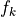could be in extremely flat region of solution space, and therefore have significant round-off errors resulting in slow or no convergence to the solution, and low overlap can also lead to underflow and overflow leading to crashed solutions.

## scipy solvers¶

pymbar is set up to use the scipy.optimize.minimize() and scipy.optimize.roots() functionality to perform this minimization. We use only the gradient-based methods, as the analytical gradient-based optimization is obtainable from the MBAR equations. Available scipy.optimize.minimize() methods include L-BFGS-B, dogleg, CG, BFGS, Newton-CG, TNC, trust-ncg, trust-krylov, trust-exact, and SLSQP. and scipy.optimize.roots options are hybr and lm. Methods that take a Hessian (dogleg, Newton-CG, trust-ncg, trust-krylov, trust-exact) are passed the analytical Hessian. Options can be passed to each of these methods through the MBAR object initialization interface.

## Built-in solutions¶

In addition to the scipy solcers, pymbar also includes an adaptive solver designed directly for MBAR. At every step, the adaptive solver calculates both the next iteration of the self-consistent iterative formula presented in Shirts et al. , and takes a Newton-Raphson step. In both cases, it calculates the gradients of the points resulting after the two steps, and selects the move that makes the magnitude of the gradient (i.e. the dot product of the gradient with itself) smallest. Far from the solution, the self-consistent iteration tends have the smaller gradient, while closer to the solution, the Newton-Raphson step tends to have the smallest gradient. It always chooses the self-consistent iteration for the first min_sc_iter iterations, as if overlap is poor then Newton=Raphson iteration can fail. min_sc_iter’s default is 2, but if one is starting from a good guess for the free energies, one could start with min_sc_iter=0

## Constructing solver protocols¶

We have found that in general, different solvers have different convergence properties and difference convergence behavior. Even at the same input tolerance level, different algorithms may not yield the same result. Additionally, accurate free energy estimates and other. Although data with states that have significant overlap in configuration states usually converge successfully with all simulation algorithms, different algorithms succeed and fail on different “hard” data sets. We have therefore constructed a very general interface to allow the user to try different algorithms.

pymbar uses the concept of a “solver protocol”, where one applies a series of methods one or multiple times. In most cases, one will never need to interact with this interface, because several different protocols have already been implemented, and are accessible with string keywords. These are currently solver_protocl=default (also the default) and solver_protocol=robust.

However, a user has the option of creating their own solver protocol. Solver protocols are created as tuples of dictionaries, where each dictionary is an optimization operation. The user has the ability to continue with the resulting free energies each time, or restarting back from the initialized free energies.

Take for example the default solver protocol, designed to give a high accuracy answer in most circumstances:

solver_protocol = (
dict(method="hybr", continuation=True),
)


Here, the first pass through optimization uses the scipy.optimize.roots function hybr, and then if it is successful, continues on by running the built-in adaptive method, but with no self-consistent iteration choices forced, as described above. If hybr fails, it will still attempted to continue on with the resulting free energies, from whatever point it ended up, issue a warning in case that adaptive is still unable to solve the result.

The options dictionary is passed onto the method, so whatever options the scipy method uses it its documentation, it can be passed on through this approach. tol is a direct option to scipy.optimize methods, and not passed on through the dictionary, and thus is passed on directly in the solver protocol.

solver_protocol = (
dict(method="hybr"),
dict(method="L-BFGS-B", tol = 1.0e-5, continuation = True, options=dict(maxiter=1000)),
dict(method="adaptive", tol = 1.0e-12, options=dict(maxiter=1000,min_sc_iter=5))
)


In this case, it would first use “hybr” with the default options and tolerance and if successful, exit. If not successful with “hybr”, it would continue on to “L-BFGS-B”, with 1000 maximum iterations, and a tolerance of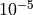but would not use the results from the “hybr” call. If “L-BFGS-B” was either successful or unsuccessful, it would pass the results to “adaptive”, where it would choose the self-consistent iteration the first five times, the numberof maximum iteractions would be 1000, and the tolerance is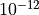.

## Initialization¶

One can initialize the soution process in a number of ways. The simplest is to start from all zeros, which is the default (and also has keyword initialize=zeros). If the keyword f_k_initial is used, then the length K.

Two other options for initialize are BAR and average-enthalpies. average-enthalpies which approximates the free energy of each state using the average enthalpy of each states, which will be valid in the limit of no entropy differences beween states. initialize=BAR can be used whenever states are given in a natural sequence of overlap, such that state 0 has the most configurational overlap with state 1, state 1 has significant configurational overlap with both states 0 and state 2, and so forth. In the limit there is only overlap between neighboring states, MBAR converges to give the same answer for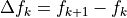that BAR gives. Although BAR also requires an iterative solution, it is a single variable problem, and thus the K-1 BAR iterations that need to be done are much faster than a single K-1 dimensional problem. The initial input for state k in the solution process is then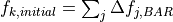Note that if both initialize and f_k_initial are selected, the logic is somewhat different. Specifing f_k_initial overwrites initialize=zeros, but initialize=BAR starts each application of BAR with the (reduced) free energy difference between states k and k+1 in from f_k_initial.

## Calculating uncertainties¶

The MBAR equations contain analytical estimates of uncertainties. These are essentially, however, the functional form is bit more complicated, since they include modifications for error propagation with implicit equations.

For free energies and expectations, one includes the analytical uncertainties by adding the keyword compute_uncertainties=True.

In some cases, to peform additional error analysis, one might need access to the covariance matrix of $ln f_k$. This is accessed in results['Theta'], and included by setting compute_theta=True, or if compute_uncertainties=True and uncertainty_method is not bootstrap.

If uncertainty_method=bootstrap, then the analytical error analysis is not performed, and instead bootstrap samples are pulled from the original distribution. Bootstrapping is done on each set of $N_k$ samples from each K states individually, rather than on the set as a whole, as the number of samples drawn from each state should not change in the bootstrapping process, or it would be a different process.

For bootstrapping to be used in calculating error estimates, the MBAR object must be initialized with the keyword n_bootstraps, which must be an integer greater than zero. In general, 50–200 bootstraps should be used to estimate uncertainties with a good degree of accuracy.

Note that users have complete control over the solver sequence for bootstrapped solutions, using the same API as for solvers of the original solution, with keyword bootstrap_solver_protocol. As an example, the default bootstrap protocol is:

bootstrap_solver_protocol = (dict(method="adaptive", tol = 1e-6, options=dict(min_sc_iter=0,maximum_iterations=100)))


The solutions for bootstrapped data should be relatively close to the solutions for the original data set; additionally, they do not need to be quite as accurate, since they are used to compute the variances.

Bootstrapped uncertainties (using uncertainty_method=bootstrap is also available for all functions calculating expectations, but again requires initialization with “n_bootstraps” when initalizing the MBAR object.